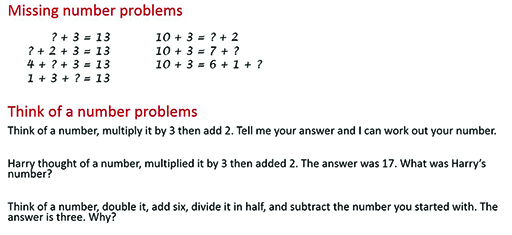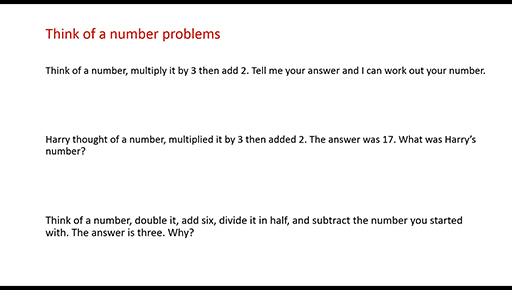Teaching mathematics

Start this free course now. Just create an account and sign in. Enrol and complete the course for a free statement of participation or digital badge if available.

Free course

# 2.2 Indeterminacy: moving from specific unknowns to variables

When learners are introduced to symbolic representations, they often represent a quantity that has a specific but unknown value. The learner may be asked to find this value.

Two examples of such problems are missing number problems and Think of a Number (THOAN) problems.

## Activity 7 Missing number problems

Timing: Allow 10 minutes

Have a go at the following problems.Figure 4 Missing number problems

## Activity 8 Reflecting

Timing: Allow 5 minutes

What makes these algebra problems, rather than simply number problems?

What do you expect learners to learn from these problems?

Now watch the two videos that discuss these questions.Skip transcript: Video 3 Missing number problems

#### Transcript: Video 3 Missing number problems

INSTRUCTOR:
In this activity, you were asked to think about what makes these algebra problems, rather than number problems, and what you expected learners to learn. Looking at the missing number problems, you can see that they involve mathematical sentences. They're similar to algebraic expressions, as they contain several terms, and they're not closed expressions.
One missing number problem on its own may involve very little algebra. For example, looking at the first one, a learner may think, question mark plus 3 equals 13-- oh, well, I know that 10 plus 3 equals 13. So the answer is 10. There's no algebra involved here. This is using a known number fact instead.
However, if you move to the second question, question mark plus 2 plus 3 equals 13, this could be done in a similar way. Or it could be done by comparison. The question mark plus 2 takes the place of the question mark in the first equation. So a question mark plus 2 is the same as 10. Question mark is 8. It's 8 plus 2 plus 3 that makes 13.
There's something to notice here about algebraic conventions. In each of these questions, in each of these equations, the question mark stands for a different value. That's quite normal in textbooks and in mathematical exercises. But when you start using algebra to model complex situations or problems, we tend to use the same letter for the same variable all the way through while working so as not to get confused.
What do we expect pupils to learn from this? Well, appreciating that you can rearrange and simplify expressions that contain unknown quantities. They could appreciate that they could compare expressions with unknown quantities. For example, we've seen a valid reasoning that the question mark in the second line is 2 less than the question mark in the first line. And they may also appreciate that the equals sign means equal in value rather than makes.
Let's look at that a bit more closely. In each set of problems, they're scoped for the learner to pay attention to generality, perhaps asking, what is the same and what is different in each set of questions. In this first set, we draw attention to the possibility of adding more than two numbers. What's the same is the answer's always 13. What's different is how many numbers are added up to make 13 and also, where the missing number appears in the different positions.
So that's how we have variation in the first set of problems. In the second set of problems, what's the same is they're all equations. And the left-hand side, 10 plus 3, is always the same. However, after the equals sign, we also have expressions that involve unknowns. And that's quite unusual for learners.
Learners expect the equal sign to give an instruction to say makes. And therefore, they expect a single term, an answer, on the right-hand side of the equals sign. So what might a learner do? They might look at 10 plus 3 equals question mark and write 10 plus 3 equals 13 and then carry on, plus 2, and give the answer 15-- question mark equals 15 as the answer there.
This would indicate an understanding of the equals sign as makes. What we're wanting them to do is understand the equals sign as equal in value. So 10 plus 3 is the same in value as 11 plus 2 since they're both equal to 13.
End transcript: Video 3 Missing number problems
Video 3 Missing number problems
Interactive feature not available in single page view (see it in standard view).Skip transcript: Video 4 THOAN

#### Transcript: Video 4 THOAN

INSTRUCTOR:
There are three versions of the think of a number problem. The second version is probably the easiest. The answer is given here. So the reasoning is to write or say a mathematical expression that represents the idea of thinking of a number, multiplying by 3, then adding 2.
So for example, we can take the idea of question mark times 3, add 2 equals 17. This could be solved simply by recalling number facts. I might know it's 5, which if you times it by 3 and add 2, it gives you 17. So the algebra is in writing the expression.
But there's also an algebraic way of solving it by focusing on undoing the sequence of operations on the number. When we do the operations, first, we times question mark by 3, then we add 2. When we undo, we start with 17, and we take them and reverse order. 17 subtract 2 is 15.
Then we undo the times 3 with a divide by 3. 15 divided by 3 is 5. So the number that Harry started with was 5. This version of the problem could be solved numerically with number facts or algebraically. And in this version of the problem, there is one specific value for the unknown. It's only 5 that gives us the answer 17.
Now, look at the first version of the problem. Here, you're just asked to think of a number and then to tell me your answer. This works quite well with the teacher asking somebody from the class and then being very quickly able to tell that person the number they first thought of. It can then be used with partners writing down and hiding their original numbers, telling each other their answers, and aiming to discover their partner's number.
Asking how many of these they can find in five minutes adds some competition to the activity. In this version, the unknown takes on a range of values, because it can be whatever the learners think of. So the algebra comes from comparing the methods used to work forwards, multiplied by 3, then adding 2, to comparing the methods used to work backwards. That's subtracting 2 and dividing by 3.
Asking the learners to work forwards themselves first gives them the experience from which they can reason about how to work backwards. Finally, in the last question here, a slightly different thing happens. You think of a number, you double it, add 6, divide in half, subtract the number you started with, and the answer is always 3, no matter what number you start with.
Here, we're getting to the idea of an unknown quantity as a variable. So how can I represent that? My number could be n. When I double it, it's 2n. I then add 6. It's 2n plus 6. I divide it in half. It's 2n plus 6 divided by 2. And I subtract the number I started with.
So I have to subtract. And I'm using n again. Subtract n, because that's the number I started with. The answer will always be 3. So I can write that as equals to 3. And the algebraic question is, why is that always true? The algebra here is used in asking learners to explain convincingly why the answer is always 3.
End transcript: Video 4 THOAN
Video 4 THOAN
Interactive feature not available in single page view (see it in standard view).
TM_1